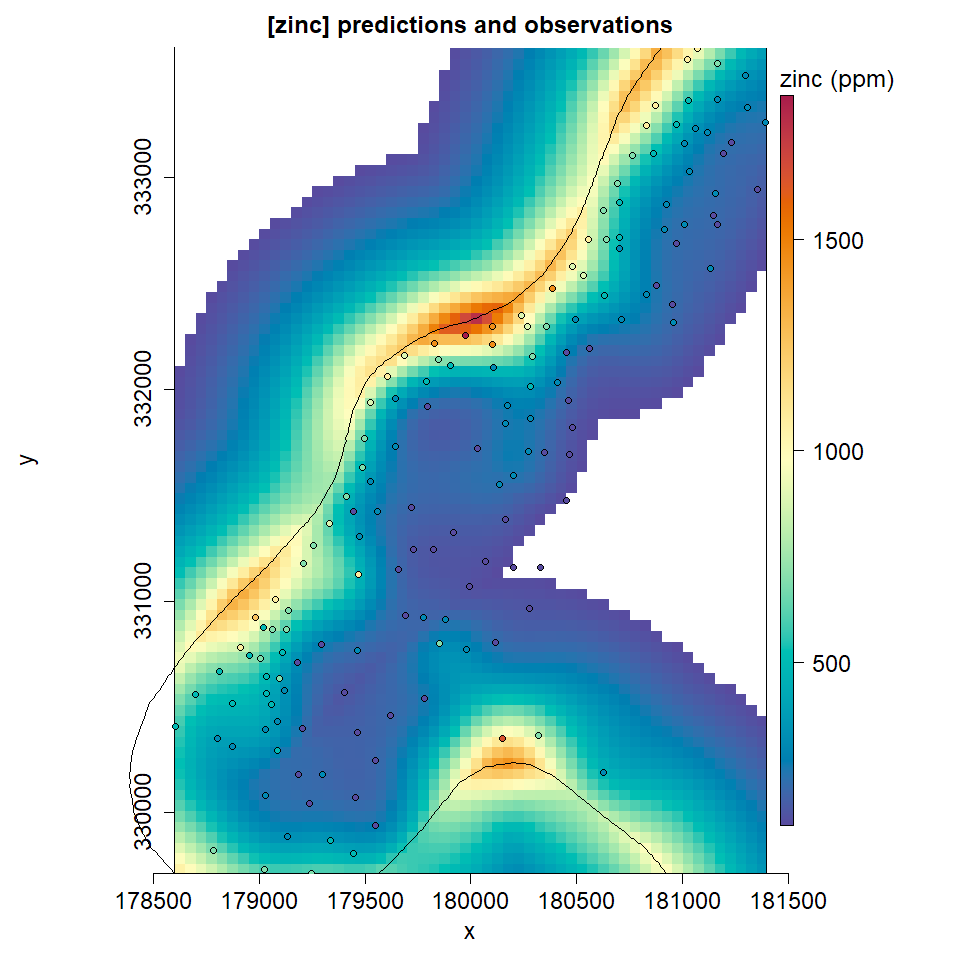# Introduction to snapKrig

## Getting started

snapKrig is for modeling spatial processes in 2-dimensions and working with associated grid data. There is an emphasis on computationally fast methods for kriging and likelihood but the package offers much more, including its own (S3) grid object class sk.

This vignette introduces the sk class and basic snapKrig functionality before showing an example of how to do universal kriging with the Meuse soils data from the sp package.

We recommend using the more up-to-date sf package to work with geo-referenced vector data, and for geo-referenced raster data we recommend terra. Both are loaded below.

library(snapKrig)

# used in examples
library(sp)
library(terra)
library(sf)
library(units)

### sk grid objects

Pass any matrix to sk to get a grid object. Start with a simple example, the identity matrix:

# define a matrix and pass it to sk to get a grid object
mat_id = diag(10)
g = sk(mat_id)

# report info about the object on console
print(g)
#> 10 x 10 complete
class(g)
#>  "sk"

An sk object stores the matrix values (if any) and the dimensions in a list, and it assigns default x and y coordinates to rows and columns. This makes it easy to visualize a matrix as a heatmap

# plot the grid with matrix theme
plot(g, ij=TRUE, main='identity matrix')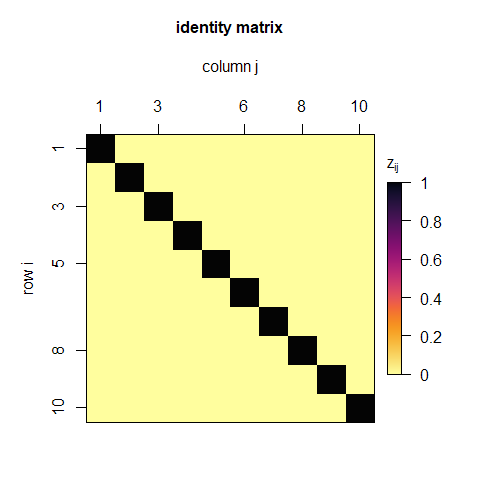sk has its own custom plot method. The result is similar to graphics::image(mat_id) except that image is not flipped and (when ij=TRUE) the axes use matrix row and column annotations instead of y and x.

snapKrig has many useful methods implemented for the sk class, including operators like + and ==

# make a grid of logical values
g0 = g == 0
print(g0)
#> 10 x 10 complete
#> (logical data)

# plot
plot(g0, ij=TRUE, col_grid='white', main='matrix off-diagonals')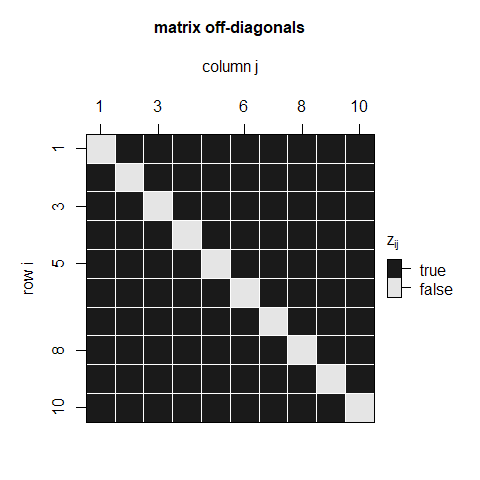The logical class prompts a gray-scale palette by default (and we added grid cell borders with col_grid). See sk_plot for more styling options.

Spatial statistics is full of large, structured matrices and I find these heatmaps helpful for getting some intuition about that structure. For example the next plot shows a covariance matrix for a square grid of points (n=100)

# get a covariance matrix for 10 by 10 grid
vmat = sk_var(sk(10))

# plot the grid in matrix style
plot(sk(vmat), ij=TRUE, main='a covariance matrix')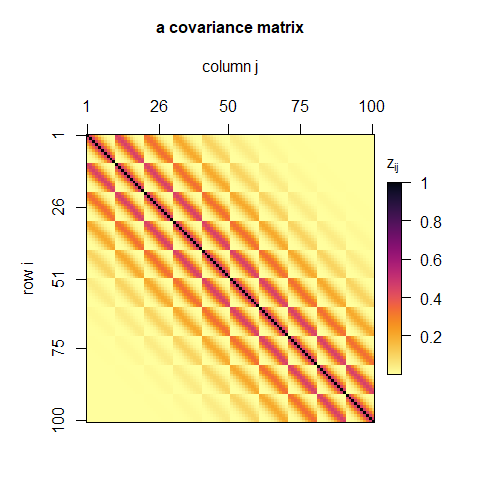Various symmetries stand out: the banding; the blocks; the Toeplitz structures - both within and among blocks; and the unit diagonal. Visualize any matrix this way. Just pass it to sk then plot.

### Vectorization

snapKrig internally stores grid data as a matrix vectorization that uses the same column-major ordering as R’s default vectorization of matrices:

# extract vectorization
vec = g[]

# compare to R's vectorization
all.equal(vec, c(mat_id))
#>  TRUE

When you pass a matrix to c or as.vector, R turns it into a vector by stacking the columns (in order). sk vectorizes in the same order, and allows square-bracket indexing, g[i], to access elements of this vector.

### Simulations

A good way to jump in and start exploring snapKrig modelling functionality is to simulate some data. This can be as simple as passing the size of the desired grid to sk_sim.

# simulate data on a rectangular grid
gdim = c(100, 200)
g_sim = sk_sim(gdim)

# plot the grid in raster style
plot(g_sim, main='an example snapKrig simulation', cex=1.5)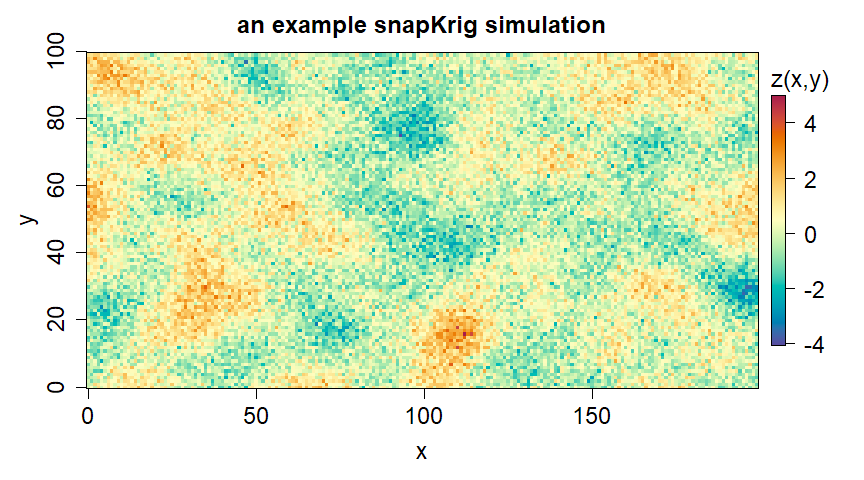You can specify different covariance models and grid layouts in sk_sim . Here is another example with the same specifications except a smaller nugget effect (‘eps’), producing a smoother output.

# get the default covariance parameters and modify nugget
pars = sk_pars(gdim)
pars[['eps']] = 1e-6

# simulate data on a rectangular grid
g_sim = sk_sim(gdim, pars)

# plot the result
plot(g_sim, main='an example snapKrig simulation (near-zero nugget)', cex=1.5)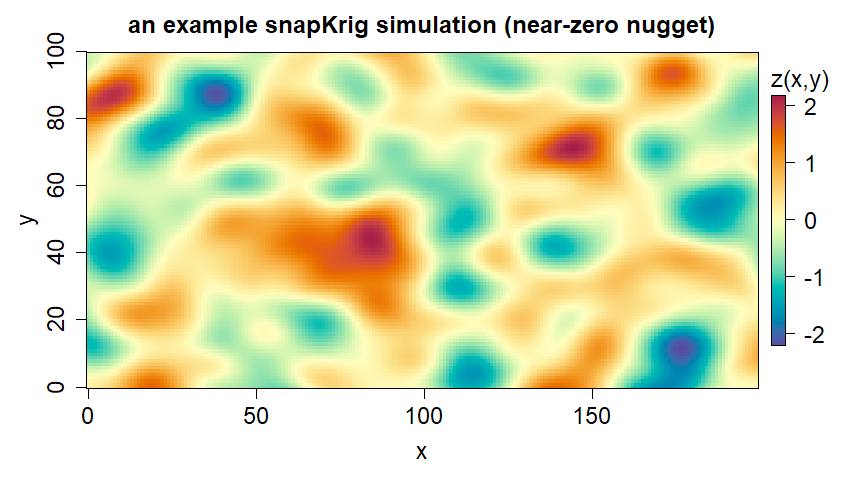snapKrig is unusually fast at generating spatially auto-correlated data like this and it supports a number of different covariance models. In simple terms, this changes the general appearance, size, and connectivity of the random blobs seen in the image above. See ?sk_corr for more on these models.

### Covariance plots

Use sk_plot_pars to visualize a covariance parameter set by showing the footprint of covariances surrounding the central point in a grid. For our simulated data, that looks like this:

# plot the covariance footprint
sk_plot_pars(pars)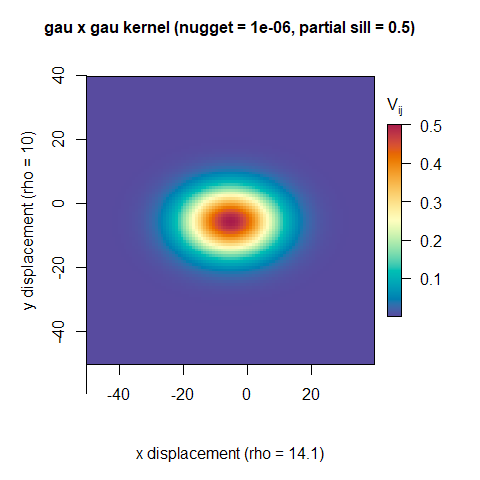### Exporting grids

The simulation plot calls above used ij=FALSE (the default), which displays the grid as a raster, much like a terra or raster layer plot call. sk grid objects are similar in content to terra’s SpatRaster object

summary(g_sim)
#> complete sk grid
#> 20000 points
#> range [-2.21, 2.16]
#> ..............................
#> dimensions : 100 x 200
#> resolution : 1 x 1
#>     extent : [0, 99] x [0, 199]

However, snapKrig functionality is more focused on spatial modeling and kriging. Outside of that context we recommend managing raster data with other packages (terra and sf in particular). sk will accept single and multi-layer rasters from the terra and raster packages, reshaping them as sk grid objects; and sk grids can be converted to SpatRaster or RasterLayer using sk_export.

sk_export(g_sim)
#> class       : SpatRaster
#> dimensions  : 100, 200, 1  (nrow, ncol, nlyr)
#> resolution  : 1, 1  (x, y)
#> extent      : -0.5, 199.5, -0.5, 99.5  (xmin, xmax, ymin, ymax)
#> coord. ref. :
#> source(s)   : memory
#> name        :     lyr.1
#> min value   : -2.213229
#> max value   :  2.163570

### Rescaling

snapKrig provides sk_rescale to change the size of a grid.

# upscale
g_sim_up = sk_rescale(g_sim, up=4)

# plot result
plot(g_sim_up, main='simulation data up-scaled by factor 4X', cex=1.5)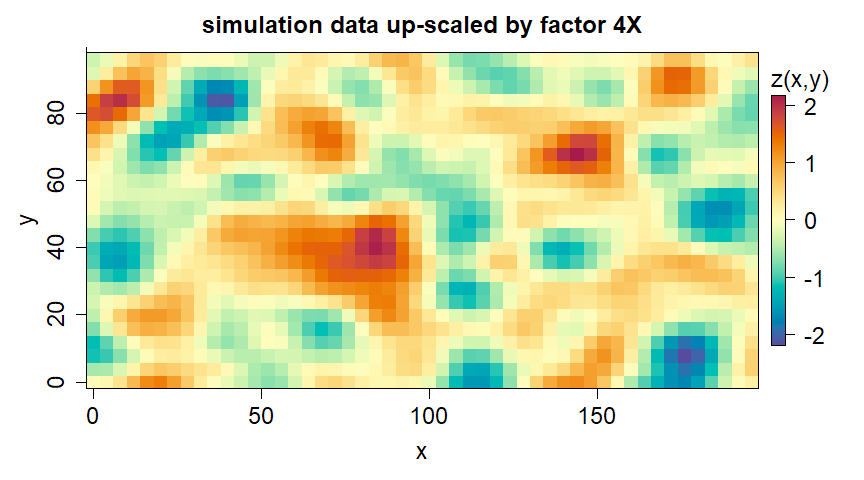Setting up=4 requests every fourth grid point along each grid line, and the rest are discarded. This results in a grid with smaller dimensions and fewer points. Setting argument down instead of up does the opposite, introducing down-1 grid lines in between each existing grid line and filling them with NAs.

# downscale
g_sim_down = sk_rescale(g_sim_up, down=4)

# plot result
plot(g_sim_down, main='up-scaled by factor 4X then down-scaled by factor 4X', cex=1.5)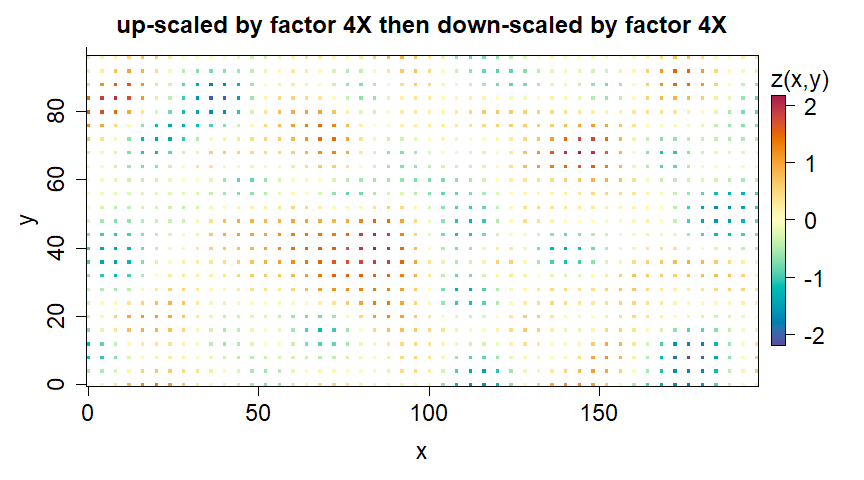This returns us to the dimensions of the original simulation grid, but we have an incomplete version now. A sparse sub-grid is observed and the rest is NA (having been discarded in the first sk_rescale call).

Down-scaling usually refers to the process of increasing grid dimensions, then imputing (guessing) values for the empty spaces using nearby observed values. sk_rescale doesn’t do imputation, but its result can be passed to sk_cmean to fill in the unobserved grid points.

# upscale
g_sim_down_pred = sk_cmean(g_sim_down, pars)
#> 25 x 50 complete sub-grid detected

# plot result
plot(g_sim_down_pred, main='down-scaled values imputed by snapKrig', cex=1.5)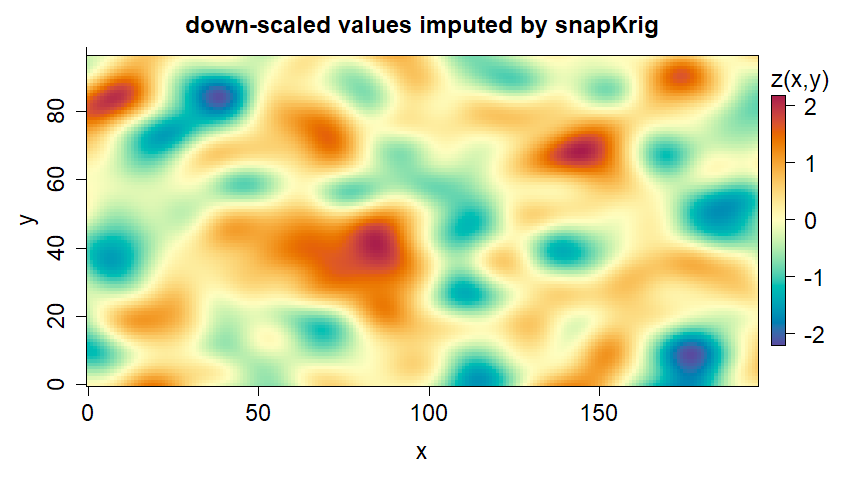sk_cmean uses conditional expectation to predict the 20,000 values in g_sim (at original resolution) based only on the 1250 observed points in g_sim_down (1/4 resolution). The function is optimized for raster data of this form (NA except for a sub-grid), and extremely fast compared to most kriging packages, making snapKrig a powerful down-scaling tool.

These results look impressive - the predictions look almost identical to our earlier plot of the full dataset (g_sim). But we are cheating here. We knew exactly which model was best for imputation (pars) because we used it to simulate the data in the first place. More often users will estimate pars from the data using maximum likelihood estimation (MLE).

### Fitting models

We recommend using MLE to fit snapKrig models. This is the process of looking for the model parameters that maximize a statistic called the likelihood, which is a function of both the parameters and the data. Roughly speaking, the likelihood scores how well the model parameters match the data.

To illustrate, consider the model (pars) that we used to generate the simulation data. Suppose the two range parameters in the model are unknown to us, but the other parameters are known. We could make a list of plausible values for the ranges and check the likelihood for each one, given the data.

# pick two model parameters for illustration
p_nm = stats::setNames(c('y.rho', 'x.rho'), c('y range', 'x range'))

# set bounds for two parameters and define test parameters
n_test = 25
bds = sk_bds(pars, g_sim_up)[p_nm, c('lower', 'upper')]
bds_test = list(y=seq(bds['y.rho', 1], bds['y.rho', 2], length.out=n_test),
x=seq(bds['x.rho', 1], bds['x.rho', 2], length.out=n_test))

To organize the results, make a grid out of the test values (similar to expand.grid) then fill it with likelihood values in a loop.

# make a grid of test parameters
g_test = sk(gyx=bds_test)
p_all = sk_coords(g_test)
#> processing 625 grid points...

# fill in the grid with log-likelihood values
for(i in seq_along(g_test))
{
# modify the model parameters with test values
p_test = sk_pars_update(pars)
p_test[p_nm] = p_all[i,]

# compute likelihood and copy to grid
g_test[i] = sk_LL(sk_pars_update(pars, p_test), g_sim_up)
}

The resulting likelihood surface is plotted below, and its maximum is circled.

# plot the likelihood surface
plot(g_test, asp=2, main='log-likelihood surface', ylab=names(p_nm), xlab=names(p_nm), reset=FALSE)

# highlight the MLE
i_best = which.max(g_test[])
points(p_all[i_best,'x'], p_all[i_best,'y'], col='white', cex=1.5, lwd=1.5)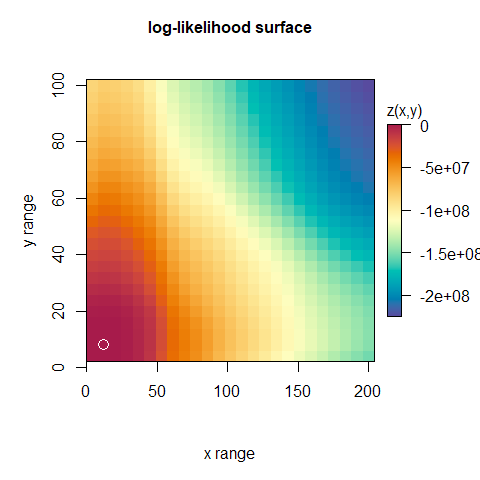This should approximately match the true scale parameter values that were used to generate the data

# print the true values
print(c(x=pars[['x']][['kp']][['rho']], y=pars[['y']][['kp']][['rho']]))
#>        x        y
#> 14.14214 10.00000

So if we didn’t know pars ahead of time (and usually we don’t), we could instead apply this principle and simply churn through plausible parameter candidates until we find the best scoring one.

However this grid search approach is usually not a very efficient way of doing MLE, and there are many good alternatives (just have a look through the CRAN’s Optimization Task View). snapKrig implements MLE for covariance models in sk_fit using stats::optim. The next section demonstrates it on a real life dataset.

## Example data: Meuse soils

This section looks at real geo-referenced points in the Meuse soils dataset (Pebesma, 2009), which reports heavy metal concentrations in a river floodplain in the Netherlands. These points are used in the kriging vignette for gstat, which we loosely follow in this vignette, and they are lazy-loaded with the sp package.

Users can access the Meuse data directly by calling data(meuse) and data(meuse.riv), which returns data frames containing coordinates. For this vignette, however, I use a helper function, get_meuse, to represent the data in a more snapKrig-friendly sf class object. The function definition for get_meuse is hidden from this document for tidiness, but it can be found in the source code (“meuse_vignette.Rmd”) just below this paragraph.

# load the Meuse data into a convenient format
meuse_sf = get_meuse()

# extract the logarithm of the zinc concentration as sf points
pts = meuse_sf[['soils']]['log_zinc']

pts is a geo-referenced sf-class points collection. This means that in addition to coordinates and data values, there is a CRS (coordinate reference system) attribute telling us how the coordinates map to actual locations on earth. This can be important for properly aligning different layers. For example, in the plot below, we overlay a polygon representing the location of the river channel with respect to the points. If this polygon had a different CRS (it doesn’t), we would have first needed to align it using sf::st_transform.

# set up a common color palette (this is the default in snapKrig)
.pal = function(n) { hcl.colors(n, 'Spectral', rev=TRUE) }

# plot source data using sf package
plot(pts, pch=16, reset=FALSE, pal=.pal, key.pos=1, main='Meuse log[zinc]')
plot(st_geometry(pts), pch=1, add=TRUE)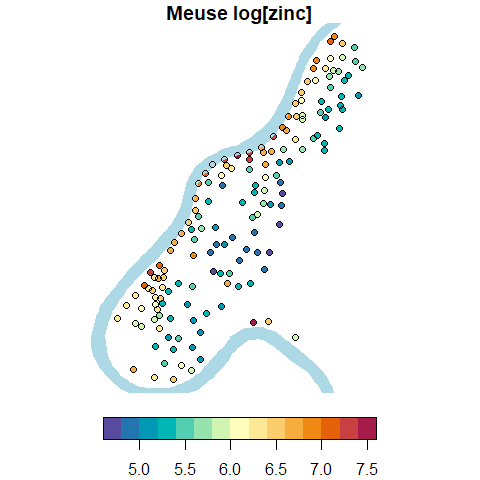### Snapping point data

snapKrig works with a regular grid representation of the data, so the first step is to define such a grid and snap the Meuse points to it using sk_snap. The extent and resolution can be selected automatically, as in…

# snap points with default settings
g = sk_snap(pts)
#> maximum snapping distance: 15.4262127060058
print(g)
#> 155 x 155 incomplete

…or they can be set manually, for example by supplying a template grid with the same CRS as pts, or by specifying some of the grid properties expected by sk. Here we will request a smaller grid by specifying a resolution of 50m by 50m

# snap again to 50m x 50m grid
g = sk_snap(pts, list(gres=c(50, 50)))
#> maximum snapping distance: 33.2640947569598
print(g)
#> 78 x 56 incomplete
summary(g)
#> incomplete geo-referenced sk grid
#> 4368 points
#> 155 observed
#> range [4.73, 7.52]
#> ..............................
#> dimensions : 78 x 56
#> resolution : 50 x 50
#>     extent : [329737.5, 333587.5] x [178622.5, 181372.5]

The units of argument ‘gres’, and of the snapping distance reported by sk_snap, are the same as the units of the CRS. This is often meters (as it is with Meuse), but if you aren’t sure you should have a look at sf::st_crs(pts) for your pts.

Call plot on the output of sk_snap to see how these points look after snapping to the grid. As with sk object plots, you can overlay additional spatial vector layers using the add argument.

# plot gridded version using the snapKrig package
plot(g, zlab='log(ppb)', main='snapped Meuse log[zinc] data')
plot(meuse_sf[['river_poly']], col='lightblue', border=NA, add=TRUE)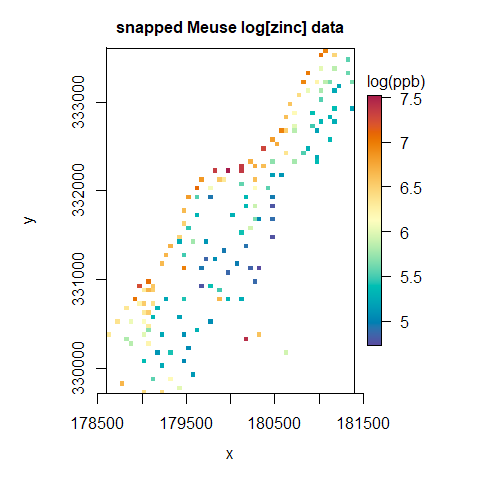Here we’ve set a fairly coarse grid resolution to keep the package build time short. The result is a somewhat pixelated-looking image and a high snapping error. This error can be controlled by reducing ‘gres’ (the spacing between grid points). Users might want to try substituting gres=c(25, 25) or gres=c(5, 5) to get a sense of the speed of snapKrig on large problems.

Be warned that if the grid resolution is fine enough, individual pixels can become invisible in plot calls, giving the false impression that there is no data. When there really is no data, the output of print(g) and summary(g) will say so. If you don’t believe them, call which(!is.na(g)) to locate the non-NAs in your grid.

### Covariates

The snapKrig model splits point values into two components: random spatial variation; and a non-random (but unknown) trend. This trend is assumed to be a linear combination of spatially varying covariates, known throughout the area of interest. The process of fitting both components of the model and then generating predictions is called universal kriging.

In this example we use just one covariate, distance to the river, but users can also supply several, or none at all (simple and ordinary kriging are also supported). snapKrig will adjust for any covariates, and fit the random spatial component to the remaining unexplained variation. This is similar to the way that we estimate variance from model residuals (observed minus fitted) in simple linear regression.

To fit a model you only need to know your covariates at the observed point locations, but to do prediction with universal kriging you will need them at all prediction locations. In our case we can create this layer directly by passing the data grid point locations and the river line geometry to sf::st_distance

# measure distances for every point in the grid
river_dist = sf::st_distance(sk_coords(g, out='sf'), meuse_sf[['river_line']])
#> processing 4368 grid points...

To create a new sk grid object containing these distances, simply copy g and replace its values with the numeric vector of distances from river_dist. We recommend also scaling all covariates for numerical stability

# make a copy of g and insert the scaled distances as grid point values
X = g
X[] = scale( as.vector( units::drop_units(river_dist) ) )
summary(X)
#> complete geo-referenced sk grid
#> 1 layer
#> 4368 points
#> range [-1.44, 3.27]
#> ..............................
#> dimensions : 78 x 56
#> resolution : 50 x 50
#>     extent : [329737.5, 333587.5] x [178622.5, 181372.5]

The result is plotted below, along with the center line of the river channel in black.

# plot the result
plot(X, zlab='distance\n(scaled)', main='distance to river covariate')
plot(meuse_sf[['river_line']], add=TRUE)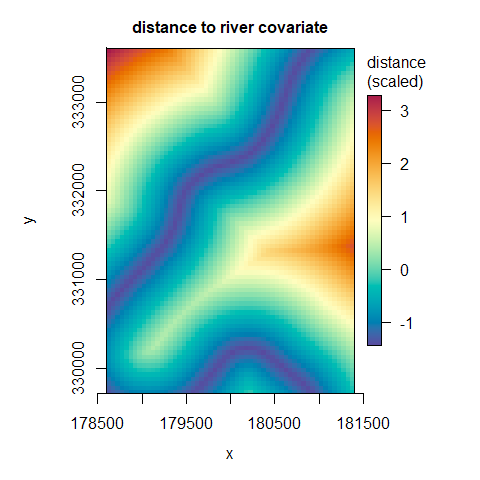It is unusual to be able to generate covariates at arbitrary locations like this. More often users will have pre-existing covariates, and their layout will dictate the layout of the prediction grid. A typical workflow therefore begins with an additional step:

1. consolidate all covariate layers into a common grid, g (possibly using terra::project)
2. snap the response data pts to this grid using sk_snap(pts, g)
3. fit the model and compute predictions

### Model fitting

For the first part of step (3) we provide sk_fit, which fits a model to data by numerical maximum likelihood. Its default settings (isotropic Gaussian covariance) will work for many applications, and they work well enough in this example. This makes model fitting very straightforward:

#fit the covariance model and trend with X
fit_result_uk = sk_fit(g, X=X, quiet=TRUE)

However, in order to get the best model fit (and the best predictions) we strongly recommend understanding and experimenting with the arguments to sk_fit. These control the covariance structure, the parameter space, and other optimizer settings. We also encourage users to check diagnostics on the parameter list returned by sk_fit using functions like sk_plot_pars and sk_plot_semi.

sk_fit fit works by searching for the maximum of the (log) likelihood function for the model given the data, using R’s stats::optim. Finding the likelihood manually for a given parameter set is simple. If the parameters are in the list form returned by sk_fit, simply pass it (along with the data and any covariates) to sk_LL.

# compute model likelihood
sk_LL(fit_result_uk, g, X)
#>  -84.04759

For users with their own preferred optimization algorithms, snapKrig also provides the convenience function sk_nLL, which is a wrapper for sk_LL that negates its result (so the problem becomes minimization), and accepts parameters in its first argument as a vector.

### Kriging

print and summary reported that g is an incomplete sk grid, and we saw from its mostly empty heatmap that the majority of the grid is unsampled (having NA grid point values). We are going to now fill in these spatial gaps using kriging predictions from sk_cmean. This is the final step of universal kriging.

# compute conditional mean and variance
g_uk = sk_cmean(g, fit_result_uk, X)

The call returns a complete version of the observed data grid g, where all values (including the observed ones) have been replaced by predictions using the model defined in fit_result_uk (returned from sk_fit), and the covariates grid(s) in X.

plot(g_uk, zlab='log[zinc]', main='universal kriging predictions')
plot(meuse_sf[['river_line']], add=TRUE)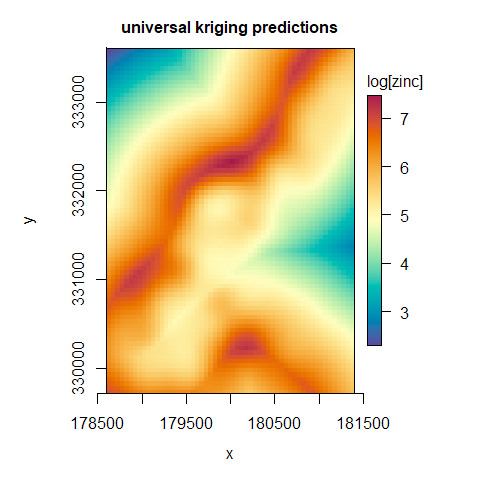We can think of this as being two images superimposed - one is the linear combination of covariates (ie the trend) and the other is the random spatial component, which is interpolated from the observed points.

In ordinary and universal kriging these two components are interrelated - the trend estimate influences the spatial component estimate and vice versa. In some special cases however, the users may wish to disentagle them (for example if the trend is known a priori, or a nonlinear trend is being modeled separately), in which case the response data (g) should be de-trended, and X should be set to 0 (not NA) in the sk_fit and sk_cmean calls. This is called simple kriging

### Uncertainty

Of all linear unbiased predictors, the kriging predictor is by definition optimal at minimizing prediction uncertainty. This is a good reason to prefer kriging, but it doesn’t mean you shouldn’t worry about uncertainty in your problem. In fact, one of the nice things about kriging theory is its explicit formula for prediction variance. We can compute it directly, rather than having to approximate.

To compute kriging variance, call sk_cmean with argument what='v'.

# compute conditional mean and variance
g_uk_var = sk_cmean(g, fit_result_uk, X, what='v', quiet=TRUE)

As before the function returns a complete grid, this time with kriging variance values. Taking square roots yields the standard error of prediction

plot(sqrt(g_uk_var), zlab='log[zinc]', main='universal kriging standard error')
plot(st_geometry(pts), pch=1, add=TRUE)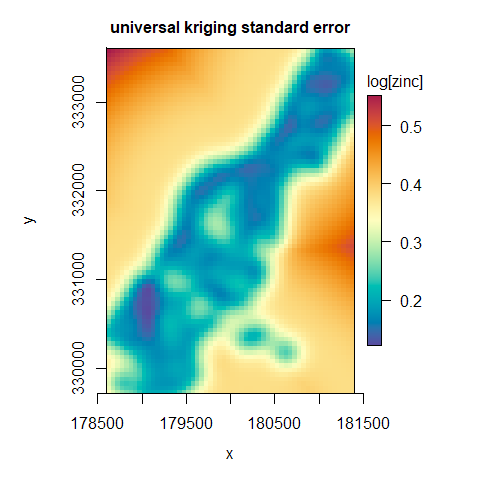The observed point locations are outlined in this plot to emphasize how uncertainty increases with distance to the nearest observation. It also increases as values of the covariates veer into extremes (locations far from the river channel), as these covariate values have no associated (zinc) observations.

Notice that even when a grid point coincides exactly with an observation, there is nonzero uncertainty. This reflects a spatially constant measurement error that is represented in the model by the nugget effect. Find this parameter in list element ‘eps’ of the parameter list returned by sk_fit.

This nugget effect is important for realism, as virtually all real-life datasets have measurement error, but it is also important for numerical stability. While it is possible to set the nugget to zero - producing an exact interpolator - this can have unpredictable results due to numerical precision issues.

### Back-transformations

So far we have been been working with the logarithms of the zinc concentrations. This produces something closer to a Gaussian random variable - a requirement of kriging theory. But when it comes to predictions and applications, we are probably after the un-transformed values.

Taking exp(g_uk), while intuitive, would introduce a negative bias. The mistake is in assuming that E(f(X)) is the same as f(E(X)) (for expected value operator E and transformation f), which is only true if f is linear.

In short, to get zinc concentration predictions on the original scale, we need a bias adjustment. We use a simplified version of the one given in Cressie (2015) - adding half the variance before exponentiating. The two plots below shows the result on its own, and again with the original observed point data overlaid.

# prediction bias adjustment from log scale
g_uk_orig = exp(g_uk + g_uk_var/2)

# points on original scale
pts_orig = meuse_sf[['soils']]['zinc']

# prediction plot
zlim = range(exp(g), na.rm=TRUE)
plot(g_uk_orig, zlab='zinc (ppm)', main='[zinc] predictions and observations', cex=1.5, zlim=zlim)
plot(meuse_sf[['river_line']], add=TRUE)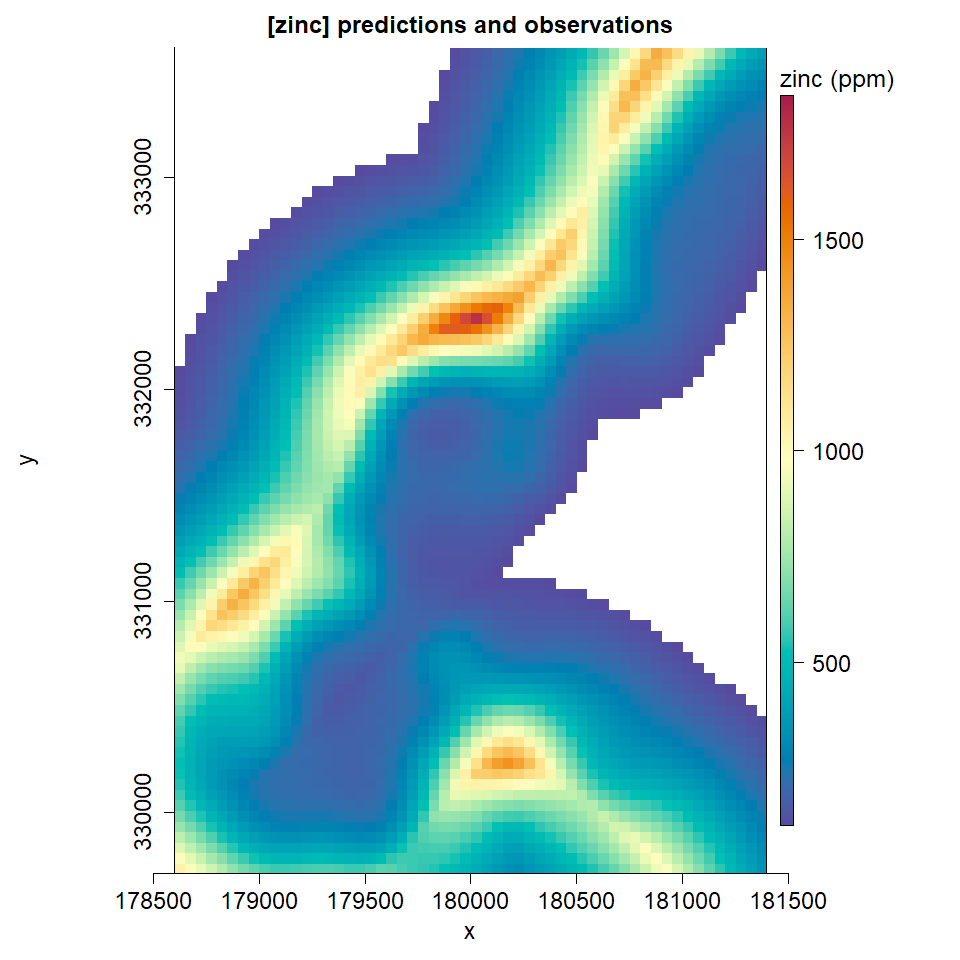# full plot
plot(g_uk_orig, zlab='zinc (ppm)', main='[zinc] predictions and observations', cex=1.5, zlim=zlim, reset=FALSE)
plot(sf::st_geometry(pts_orig), add=TRUE)